Search

Proving Trigonometric Identities (page 1 of 3)Proving an identity is very different in concept from solving an equation. Though you'll use many of the same techniques, they are not the same, and the differences are what can cause you problems.

An "identity" is a tautology, an equation or statement that is always true, no matter what. For instance, sin(x) = 1/csc(x) is an identity. To "prove" an identity, you have to use logical steps to show that one side of the equation can be transformed into the other side of the equation. You do not plug values into the identity to "prove" anything. There are infinitely-many values you can plug in. Are you really going to "prove" anything by listing three or four values where the two sides of the equation are equal? Of course not. And sometimes you'll be given an equation which is not an identity. If you plug a value in where the two sides happen to be equal, such as π/4 for the (false) identity sin(x) = cos(x), you could fool yourself into thinking that a mere equation is an identity. You'll have shot yourself in the foot. So let's don't do that.

To prove an identity, your instructor may have told you that you cannot work on both sides of the equation at the same time. This is correct. You can work on both sides together for a regular equation, because you're trying to find where the equation is true. When you are working with an identity, if you work on both sides and work down to where the sides are equal, you will only have shown that, if the starting equation is true, then you can arrive at another true equation. But you won't have proved, logically, that the original equation was actually true.

Since you'll be working with two sides of an equation, it might be helpful to introduce some notation, if you haven't seen it before. The "left-hand side" of an equation is denoted by LHS, and the "right-hand side" is denoted as RHS.

• Prove the identity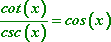• It's usually a safe bet to start working on the side that appears to be more complicated. In this case, that would be the LHS. Another safe bet is to convert things to sines and cosines, and see where that leads. So my first step will be to convert the cotangent and cosecant into their alternative expressions: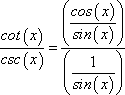Now I'll flip-n-multiply: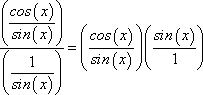Now I can see that the sines cancel, leaving me with: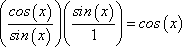Then my proof of the identity is all of these steps, put together: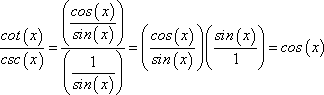That final string of equations is what they're wanting for your answer.

• Prove the identity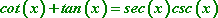• I'm not sure which side is more complicated, so I'll just start on the left. My first step is to convert everything to sines and cosines: Copyright © Elizabeth Stapel 2010-2011 All Rights Reserved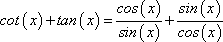When I get fractions, it's almost always a good idea to get a common denominator, so I'll do that next: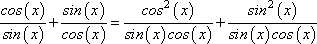Now that I have a common denominator, I can combine these fractions into one: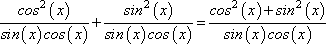Now I notice a Pythagorean identity in the numerator, allowing me to simplify: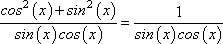Looking back at the RHS of the original identity, I notice that this denominator could be helpful. I'll split the product into two fractions: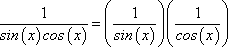And now I can finish up by converting these fractions to their reciprocal forms: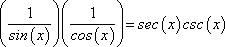(I wrote them in the reverse order, to match the RHS.) The complete answer is all of the steps together, starting with the LHS and ending up with the RHS: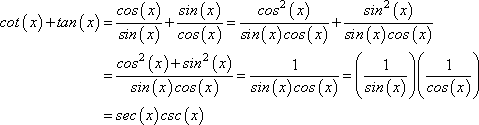Top  |  1 | 2 | 3  |  Return to Index  Next >>

 Cite this article as: Stapel, Elizabeth. "Provinig Trigonometric Identities." Purplemath. Available from     https://www.purplemath.com/modules/proving.htm. Accessed [Date] [Month] 2016

Study Skills Survey

Tutoring from Purplemath
Find a local math tutor